Function Repository Resource:

# InverseStereographicProjection

Compute the parametrization of a curve projected onto the unit sphere

Contributed by: Wolfram Staff (original content by Alfred Gray)
 ResourceFunction["InverseStereographicProjection"][{u,v}] projects {u,v} from a plane onto the unit sphere.

## Details and Options

The projection obtained is generated by projecting points lying in a plane tangent to the south pole of a sphere to the sphere's north pole and plotting the intersection of the projection segment with the sphere.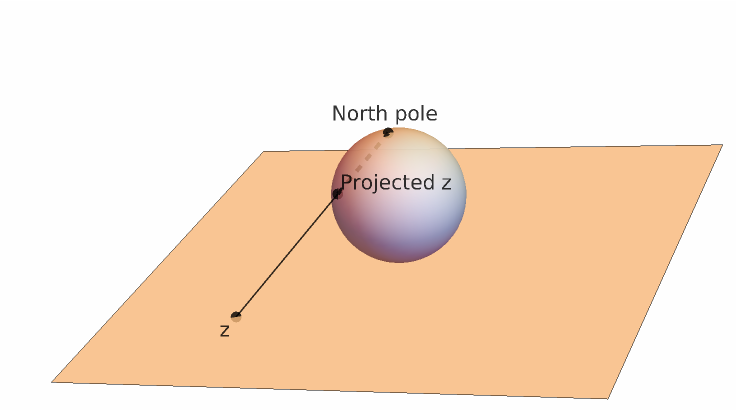## Examples

### Basic Examples (1)

Compute the inverse stereographic projection of a generic point:

 In:=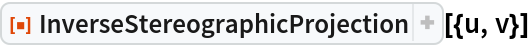Out=### Scope (3)

Define an ellipse:

 In:=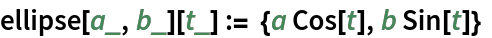Project an ellipse onto the sphere:

 In:=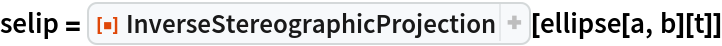Out=Plot the projected ellipse:

 In:=Out=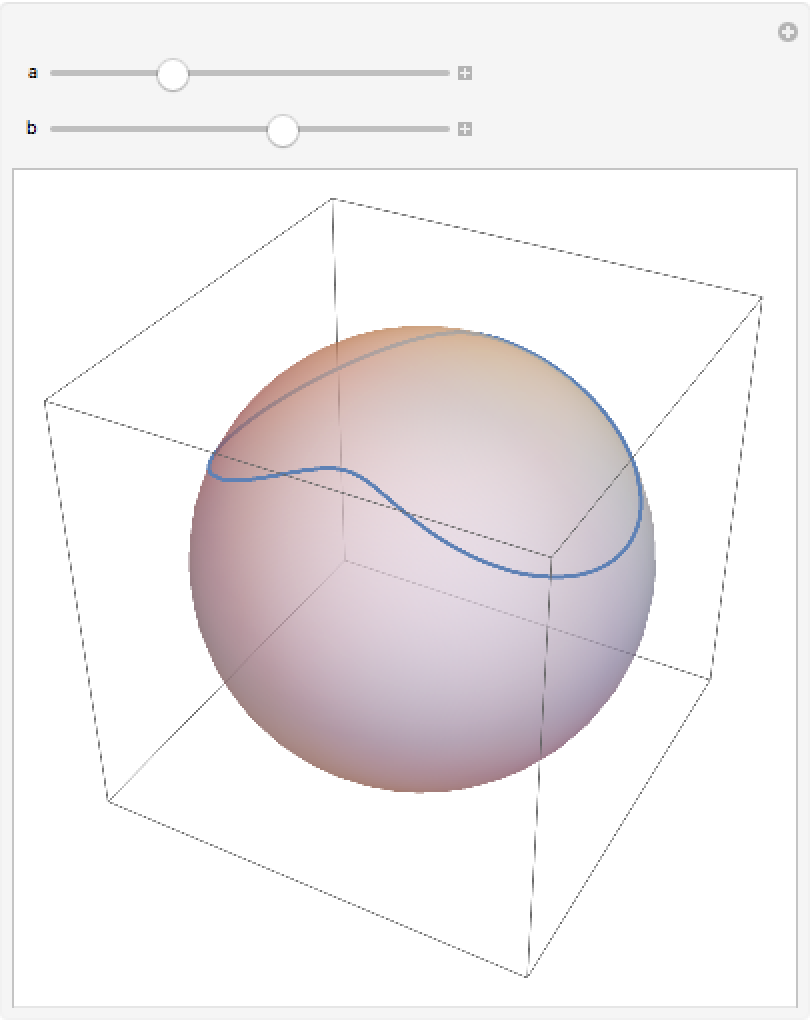Define a logarithmic spiral curve:

 In:=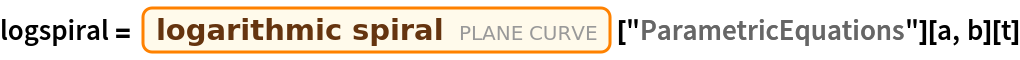Out=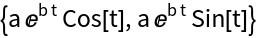A plot of the logarithmic spiral:

 In:=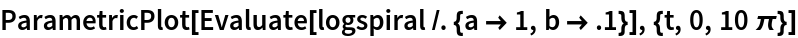Out=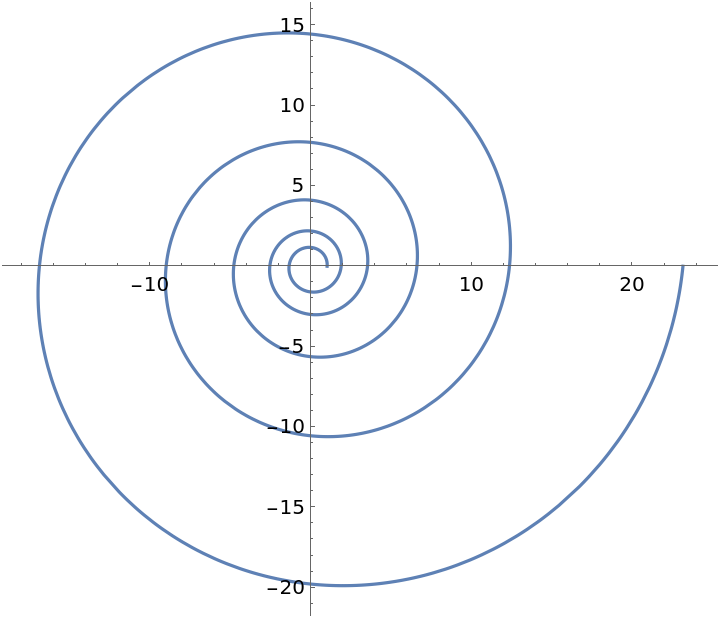Project the spiral onto the sphere; it becomes a spherical loxodrome:

 In:=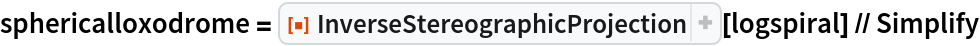Out=Compute the norm of the logarithmic spiral:

 In:=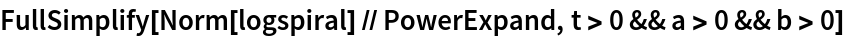Out=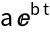Plot the projected spiral with meridians:

 In:=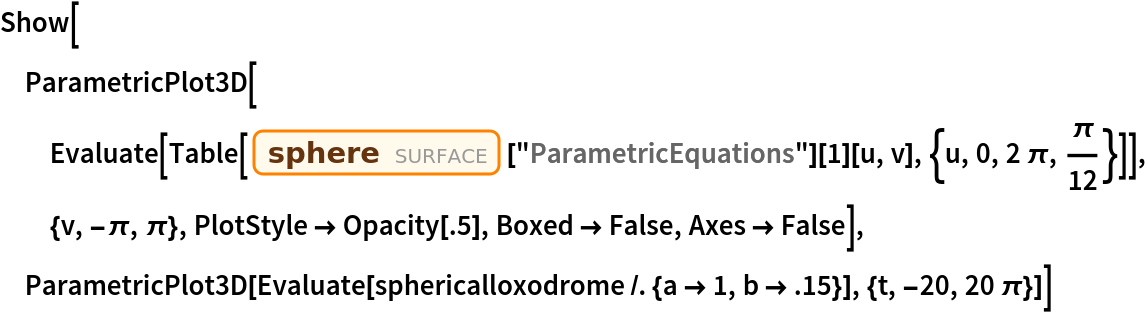Out=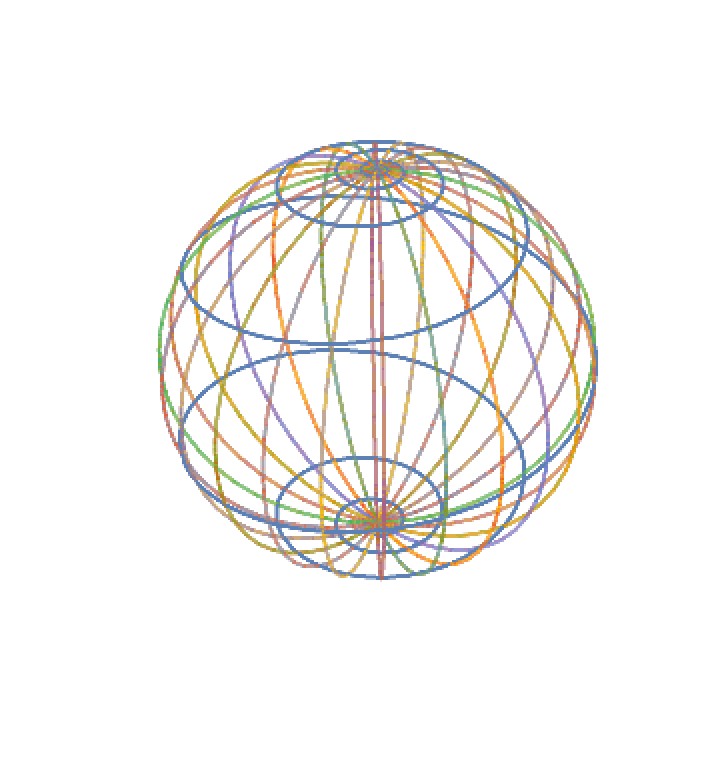Plot the spherical loxodrome:

 In:=Out=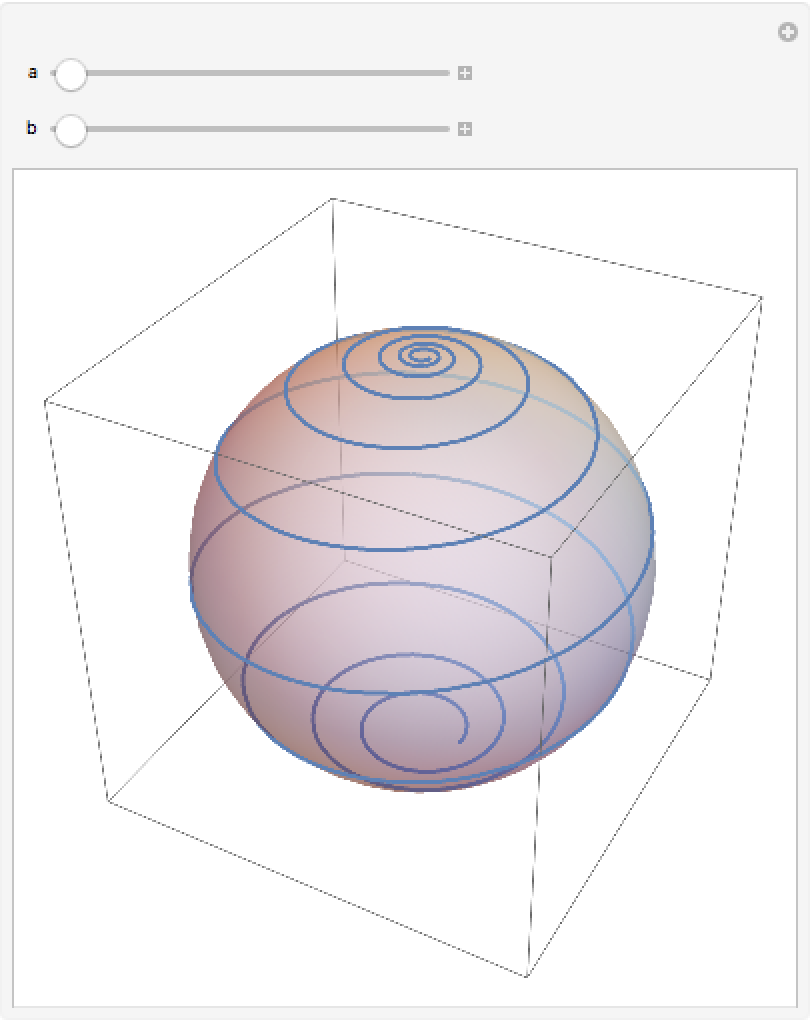Define the second butterfly curve:

 In:=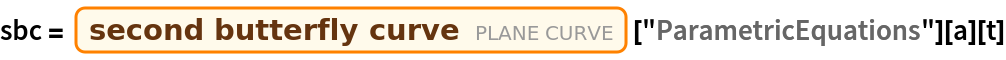Out=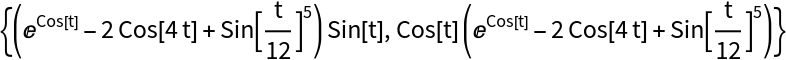Project the curve onto the sphere:

 In:=Out=Plot the curve and the projection:

 In:=Out=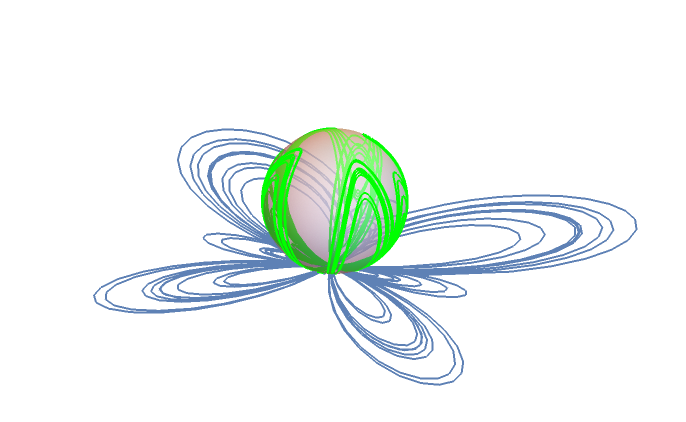### Properties and Relations (3)

The norm of the stereographic sphere:

 In:=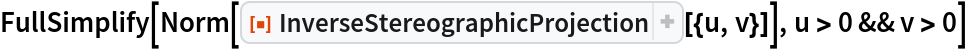Out=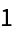Project a grid of points in Cartesian coordinates:

 In:=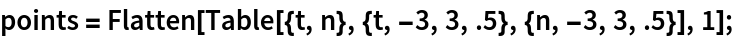A Cartesian grid on the plane appears distorted on the sphere; show the points and their projections onto the sphere:

 In:=Out=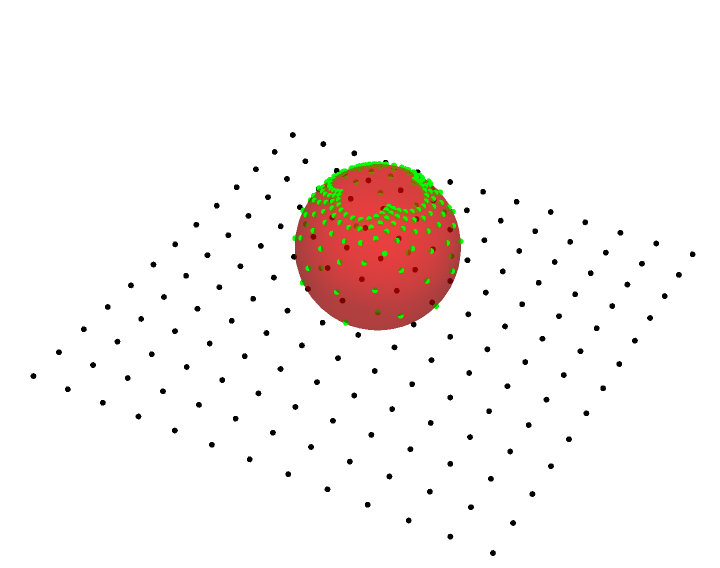Project a grid of lines instead:

 In:=Show the lines and their projections:

 In:=Out=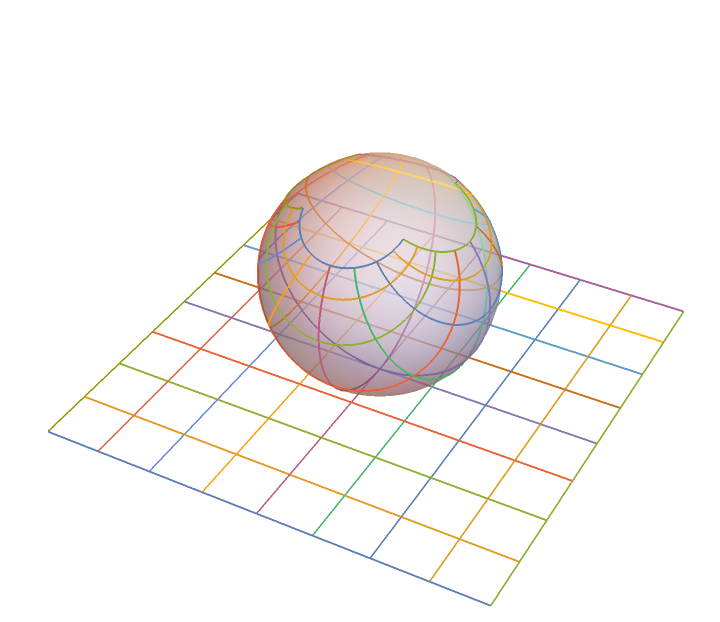The same mesh can be generated by ParametricPlot3D. The grid lines are still perpendicular, but the sectors get smaller close to the north pole:

 In:=Out=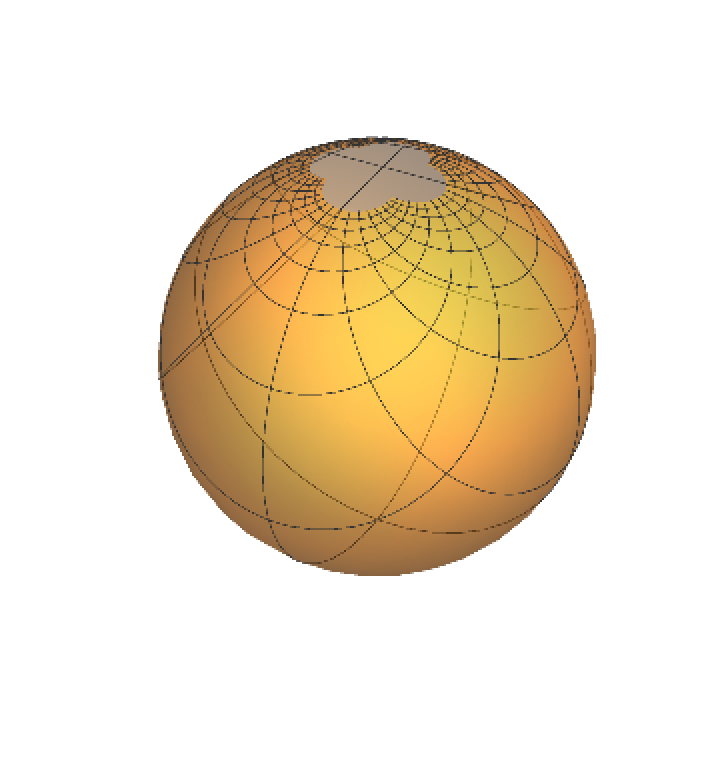Enrique Zeleny

## Version History

• 1.0.0 – 05 June 2020